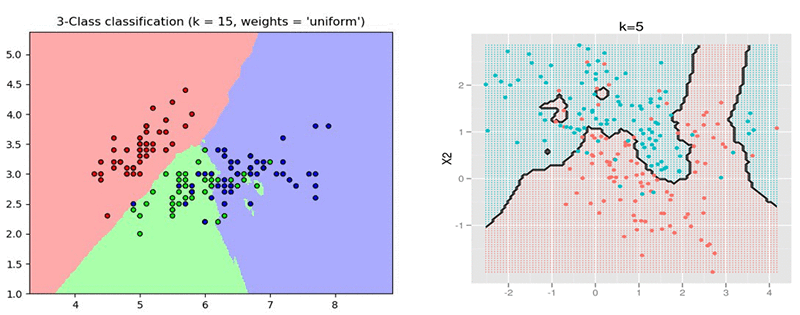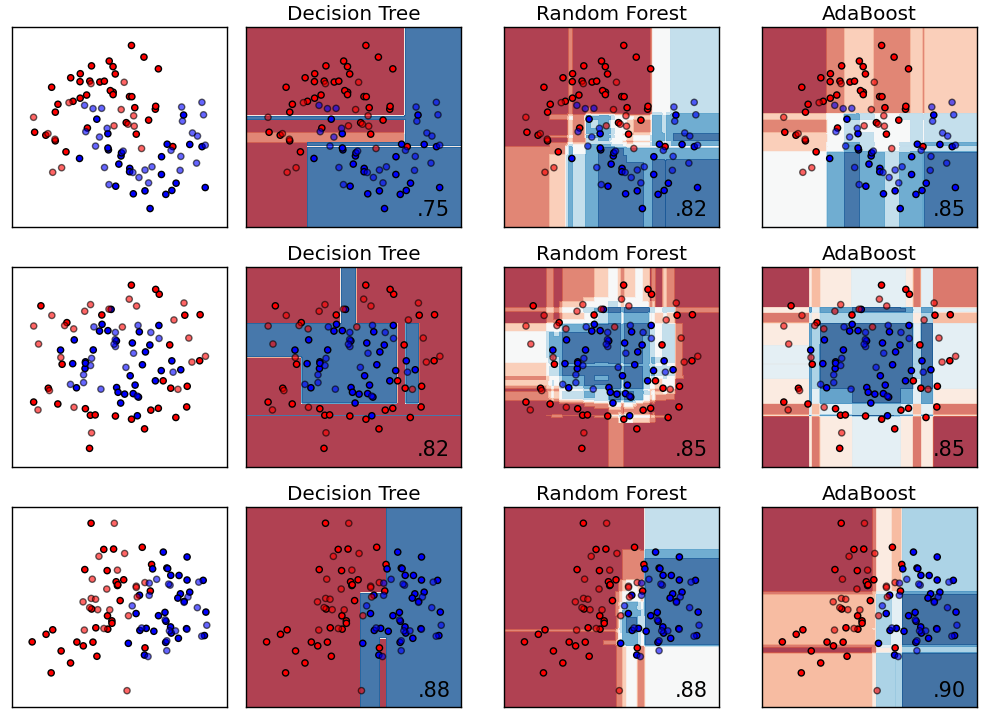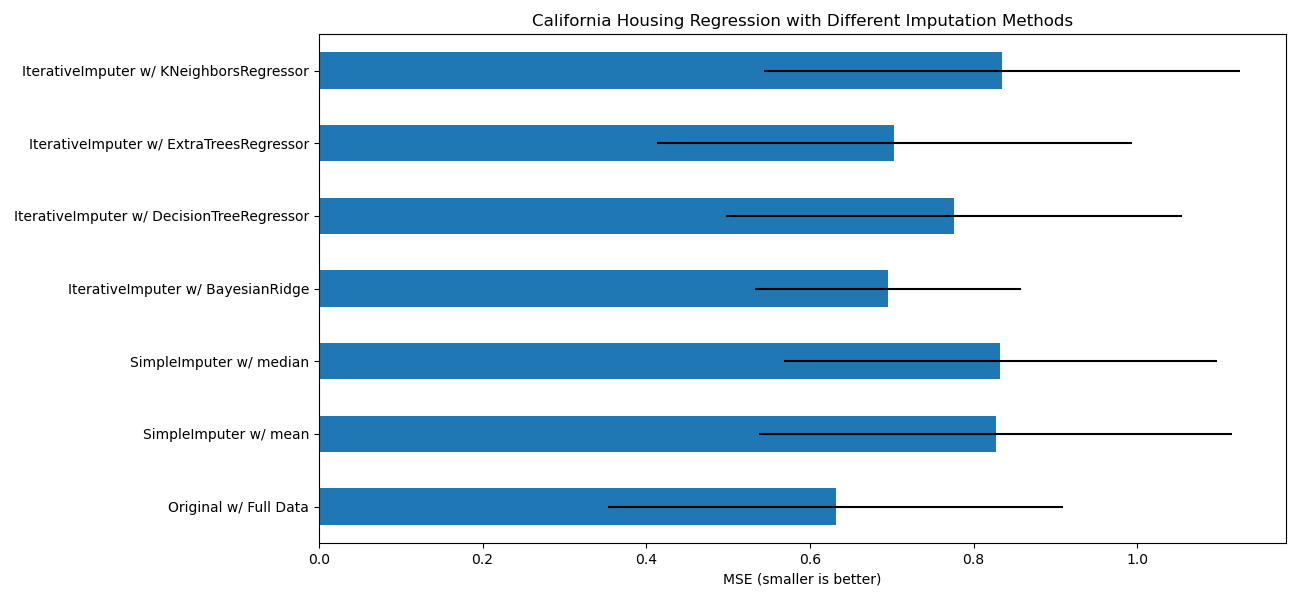# ML23: Handling Missing Values

## Approaches besides imputing mean, median or mode

Common ways of dealing with missing values include dropping as well as imputing mean, median or mode. Also, there are advanced approaches like Random Forest & BayesianRidge. Then, how to ensure the best practice of processing missing values avoiding underfitting & overfitting?

# (1) Prerequisites

## 1–1 Terms 

1. NA = A missing value. Stands for not available or not applicable.

The way that missing data is represented in pandas objects is somewhat imperfect, but it is functional for a lot of users. For numeric data, pandas uses the floating-point value NaN (Not a Number) to represent missing data. We call this a sentinel value that can be easily detected:

In pandas, we’ve adopted a convention used in the R programming language by referring to missing data as NA, which stands for not available. In statistics applications, NA data may either be data that does not exist or that exists but was not observed (through problems with data collection, for example). When cleaning up data for analysis, it is often important to do analysis on the missing data itself to identify data collection problems or potential biases in the data caused by missing data. 

2. feature = ML-specific term, also called predictor, independent variable, explanatory variable, regressor, exogenous or input in Statistics and Economics.
3. target = ML-specific term, also called response, dependent variable, regressand, endogenous or output in Statistics and Economics.
4. data point = ML-specific term, also called observation or case in Statistics. A data point is a single row of a dataframe.
5. dataset/dataframe = Lots of data points comprise a dataset/dataframe.

## 1–2 Data Type 

In general, we classify the data into two categories:

[i] Numeric data: continuous & discrete
[ii] Categorical data: ordinal & nominal

Further, we may sort the data into:

[i] Numeric data: ratio level & interval level
[ii] Categorical data: ordinal level & nominal level

Then, let’s probe into these four levels of data:

1. Ratio level: Can be classified, sorted as well as added, subtracted, multiplied and divided. E.g., income.
2. Interval level: Can be classified, sorted as well as added, subtracted. Can’t be multiplied and divided. E.g., degrees Celsius. This type of data is rare, only degrees Celsius, degrees Fahrenheit, and few Likert Scale.
3. Ordinal level: Can be classified, sorted. Can’t be added, subtracted, multiplied and divided. E.g., a question of a survey with 5 options, 1~5 points respectively.
4. Nominal level: Can be classified only. E.g., colors of cars.

# (2) Two Kinds of Missing Values 

In fact, there are three kinds of them: missing completely at random (MCAR), missing at random (MAR) & missing not at random (MNAR) . But for simplicity, we focus on two kinds of them: missing randomly & missing systematically, that is, MCAR & MNAR respectively.

Here comes the question: How to identify NAs as MCAR or MNAR? Zumel and Mount (2014) indicates that:

“If you don’t know whether the missing values are random or systematic, we recommend assuming that the difference is systematic, rather than trying imputing values to the variables based on the faulty sensor assumption.”

In short, without loss of generality, we should assume NAs to be MNAR. Based on this recommendation, we now dig into numeric data and categorical data.

{A} Categorical data: Assuming NAs are MNAR, we can simply classify all NAs into a special category.

{B} Numeric data: Assuming NAs are MNAR, we then have few options and those methods would drastically reduce the information the numeric data contain, e.g., transforming numeric data into category data and do the procedure above. Hence, in practice, we often assume NAs in numeric data are missing randomly to some extent and take them as MCAR, then we can impute the NAs with mean, median, mode or other ML methods like BayesianRidge & Random Forest.

# (3) Methods of Handling Missing Values

Let’s walk through missing value imputation methods. Keep in mind that most of the methods assume NAs are missing randomly to some extent and can be seen as MCAR.

## 1. Dropping NAs

It’s the easiest and most intuitive method, deleting all data points (all rows) containing any NA. Yet it’s a devastating way since it slashes the information of the dataset and generates bias in the dataset.

## 2. Mean/Median/Mode Imputation

In contrast to dropping all data points containing any NA, this method is better. Some drawbacks are reducing variances, twisting distributions and lessening the observed relations.

[A] Numeric data: mean/median imputation.

[B] Categorical data: mode imputation.

## 3. Hot Deck Imputation

Pretty much like kNN . Better than mean/median/mode imputation on average.

## 4. Regression Imputation

[A] Advantages: More precise than mean/median/mode imputation.

[B] Disadvantages: Underestimating variances, reinforcing existed relations, reducing generalizations, variables must have correlation to yield valid values, imputed values might be out of reasonable ranges.

## 5. Multiple Imputation

Multiple imputation is a general approach to the problem of missing data that is available in several commonly used statistical packages. It aims to allow for the uncertainty about the missing data by creating several different plausible imputed data sets and appropriately combining results obtained from each of them. 

## 6. ML Algorithm Imputation

kNN, Decision Tree, Random Forest, BayesianRidge and so forth.

## 7. Other Imputation Methods

7–1 Creating a “NAs” Category

As mentioned in previous paragraph, if we identify the NAs are MNAR, we can address NAs of categorical data & numeric data as follows:

{A} Categorical data: Assuming NAs are MNAR, we can simply classify all NAs into a special category.

{B} Numeric data: Assuming NAs are MNAR, we then have few options and those methods would drastically reduce the information the numeric data contain, e.g., transforming numeric data into category data and do the procedure above. Hence, in practice, we often assume NAs in numeric data are missing randomly to some extent and take them as MCAR, then we can impute the NAs with mean, median, mode or other ML methods like BayesianRidge & Random Forest.

7–2 Converting NAs into a Certain Number

This is for numeric data. If you are able to discover some kind of insight, for instance, those people with feature “income” missing are mostly housewife and students, then we might reasonably convert these NAs into “0”. Further, we can create a new feature “income_NA” (called masking variable), classifying those with “income” missing as “1” and the rest as “0”.

# (4) Imputation in Practice

Moving on, we now take a closer look at missing values imputation methods in practice from different sources.

## 4–1 Dropping NAs & Mean/Median/Mode Imputation

Though handling missing values is one of prominent procedure in data preprocessing, many popular Python & R books like McKinney (2018), VanderPlas (2017), Wickham and Grolemund (2016) and James G. et al. (2013)  only talk about dropping NAs, mean/median/mode imputation and interpolation imputation with no further methods mentioned.

## 4–2 kNNFigure 1: Decision boundaries of kNN 

…we can use machine learning to predict the values of the missing data. To do this we treat the feature with missing values as a target vector and use the remaining subset of features to predict missing values. While we can use a wide range of machine learning algorithms to impute values, a popular choice is KNN. 

Albon(2018)  points out that kNN is a popular choice for missing value imputation. Nevertheless, as far as I am concerned, kNN is a lazy learning way, which is time-consuming, that only fit in with small-size dataset. Moreover, I don’t reckon kNN would outperform RF, which is more efficient and work alike, on average referring to the decision boundaries of kNN, SVM(Linear), SVM (RBF), Decision Tree, Random Forest, AdaBoost depicted in the following two figures.Figure 2 & 3: Decision boundaries of different models 

## 4–3 ML Imputation Methods

The documentation of sklearn.impute.IterativeImputer introduce a test measuring MSE of all kinds of imputation methods on notable “California housing” dataset . The following resulting ranking showcases unexpected findings:

BayesianRidge ≈ ExtraTreesRegressor > DecisionTreeRegressor > KNeighborsRegressor ≈ mean ≈ medianFigure 4: Comparison of imputation methods 
1. BayesianRidge: This can be viewed as Bayesian multiple imputation + regularization term ( lasso & ridge) according to the documentation.
2. ExtraTreesRegressor: It’s similar to missForest( ) in R. Additionally, missForest( ) is close to mice( ) using rf; however, documentation of missForest( ) indicates “It can be run in parallel to save computation time.” Hence, missForest( ) can be viewed as efficient version of mice( ) using rf.
3. DecisionTreeRegressor: Decision tree.
4. KNeighborsRegressor: kNN.

# (5) Conclusion

In Python, BayesianRidge & ExtraTreesRegressor of sklearn.impute.IterativeImputer might be the best choices for imputing missing values. In R, missForest( ) in package missForest & Bayesian-related methods in packages mice or VIM are must-try methods.

# (6) References

 McKinney, W. (2018). Python for Data Analysis: Data Wrangling with Pandas, NumPy, and IPython. California, CA: O’Reilly Media.

 Cheng, T. C. (Unidentified). Handout of Graduate Level Course Applied Regression Analysis of department of Statistics in NCCU.

 Ozdemir, S., & Susarla, D. (2018). Feature Engineering Made Easy. Birmingham, UK: Packt.

 Albon, C. (2018). Machine Learning with Python Cookbook: Practical Solutions from Preprocessing to Deep Learning. California, CA: O’Reilly Media.

 Zumel, N., & Mount, J. (2014). Practical Data Science with R. Shelter Island, NY: Manning.

 Kowarik, A., & Templ, M. (2016). Imputation with the R Package VIM. Journal of Statistical Software, 74(7). Retrieved from https://bit.ly/3cqv7Qy

 Sterne, J.A.C., R White I.R. et al. (2009). Multiple imputation for missing data in epidemiological and clinical research: potential and pitfalls. Retrieved from https://bit.ly/30EFmv6

 VanderPlas, J. (2017). Python Data Science Handbook: Essential Tools for Working with Data. California, CA: O’Reilly Media.

 Wickham, H., & Grolemund, G. (2016). R for Data Science. California, CA: O’Reilly Media.

 James, G. et al. (2013). An introduction to Statistical learning: with Applications in R. New York, NY: Springer.

 Amazon (2018). Amazon SageMaker supports kNN classification and regression. Retrieved from https://amzn.to/2Oy6nhp

 Same as 

 Thoma, M. (2016). Comparing Classifiers. Retrieved from https://bit.ly/2OPx5lw

 scikit-learn.org (Unidentified). Imputing missing values with variants of IterativeImputer. Retrieved from https://bit.ly/3qLx4fu

--

--